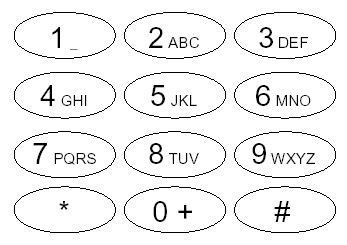# POJ 1451 T9（Trie+思维）

### Description

Background

A while ago it was quite cumbersome to create a message for the Short Message Service (SMS) on a mobile phone. This was because you only have nine keys and the alphabet has more than nine letters, so most characters could only be entered by pressing one key several times. For example, if you wanted to type "hello" you had to press key 4 twice, key 3 twice, key 5 three times, again key 5 three times, and finally key 6 three times. This procedure is very tedious and keeps many people from using the Short Message Service.

This led manufacturers of mobile phones to try and find an easier way to enter text on a mobile phone. The solution they developed is called T9 text input. The "9" in the name means that you can enter almost arbitrary words with just nine keys and without pressing them more than once per character. The idea of the solution is that you simply start typing the keys without repetition, and the software uses a built-in dictionary to look for the "most probable" word matching the input. For example, to enter "hello" you simply press keys 4, 3, 5, 5, and 6 once. Of course, this could also be the input for the word "gdjjm", but since this is no sensible English word, it can safely be ignored. By ruling out all other "improbable" solutions and only taking proper English words into account, this method can speed up writing of short messages considerably. Of course, if the word is not in the dictionary (like a name) then it has to be typed in manually using key repetition again.Figure 8: The Number-keys of a mobile phone.

More precisely, with every character typed, the phone will show the most probable combination of characters it has found up to that point. Let us assume that the phone knows about the words "idea" and "hello", with "idea" occurring more often. Pressing the keys 4, 3, 5, 5, and 6, one after the other, the phone offers you "i", "id", then switches to "hel", "hell", and finally shows "hello".

Problem

Write an implementation of the T9 text input which offers the most probable character combination after every keystroke. The probability of a character combination is defined to be the sum of the probabilities of all words in the dictionary that begin with this character combination. For example, if the dictionary contains three words "hell", "hello", and "hellfire", the probability of the character combination "hell" is the sum of the probabilities of these words. If some combinations have the same probability, your program is to select the first one in alphabetic order. The user should also be able to type the beginning of words. For example, if the word "hello" is in the dictionary, the user can also enter the word "he" by pressing the keys 4 and 3 even if this word is not listed in the dictionary.

### Input

The first line contains the number of scenarios.

Each scenario begins with a line containing the number w of distinct words in the dictionary (0<=w<=1000). These words are iven in the next w lines in ascending alphabetic order. Every line starts with the word which is a sequence of lowercase letters from the alphabet without whitespace, followed by a space and an integer p, 1<=p<=100, representing the probability of that word. No word will contain more than 100 letters.

Following the dictionary, there is a line containing a single integer m. Next follow m lines, each consisting of a sequence of at most 100 decimal digits 2�, followed by a single 1 meaning "next word".

### Output

The output for each scenario begins with a line containing "Scenario #i:", where i is the number of the scenario starting at 1.

For every number sequence s of the scenario, print one line for every keystroke stored in s, except for the 1 at the end. In this line, print the most probable word prefix defined by the probabilities in the dictionary and the T9 selection rules explained above. Whenever none of the words in the dictionary match the given number sequence, print "MANUALLY" instead of a prefix.

Terminate the output for every number sequence with a blank line, and print an additional blank line at the end of every scenario.

### Sample Input

2
5
hell 3
hello 4
idea 8
next 8
super 3
2
435561
43321
7
another 5
contest 6
give 13
integer 6
new 14
program 4
5
77647261
6391
4681
26684371
77771

### Sample Output

Scenario #1:
i
id
hel
hell
hello

i
id
ide
idea

Scenario #2:
p
pr
pro
prog
progr
progra
program

n
ne
new

g
in
int

c
co
con
cont
anoth
anothe
another

p
pr
MANUALLY
MANUALLY

const int N=1e5+5;

int n,m;
int i,j,k;
char s[N];
int t[N],tot=0,sum[N];
int bel;
map<string,string> mp;
map<string,int> maxx;

int idx(char ch){ return ch-'a'; }

void insert(char *s,int c)
{
int rt=0;
string str="",res="";
for(int i=0;s[i];i++){
int x=idx(s[i]);
res+=(bel[x]+'0'); //手机按键号码
str+=s[i]; //原字符串前缀
if(!t[rt][x]) t[rt][x]=++tot;
rt=t[rt][x];
sum[rt]+=c;
if(sum[rt]>maxx[res]){
mp[res]=str;
maxx[res]=sum[rt];
}
//dbg(res);
}
}

void clear()
{
mp.clear(),maxx.clear();
for(int i=0;i<=tot;i++){
sum[i]=0;
for(int j=0;j<26;j++) t[i][j]=0;
}
}

int main()
{
//IOS;
int num=0;
for(int i=2;i<=9;i++){
if(i==7 || i==9) {
for(int j=0;j<4;j++) bel[num++]=i;
} else{
for(int j=0;j<3;j++) bel[num++]=i;
}
}
//for(int i=0;i<26;i++) dbg(bel[i]);
num=0;
rush(){
sd(n);
for(int i=1;i<=n;i++){
int x;
ss(s); sd(x);
insert(s,x);
}
string res;
sd(m);
printf("Scenario #%d:\n",++num);
for(int i=1;i<=m;i++){
ss(s);
res="";
int len=strlen(s);
for(int i=0;i<len-1;i++){
res+=s[i];
if(maxx[res]) ps(mp[res].c_str());
else puts("MANUALLY");
}
puts("");
}
clear();
puts("");
}
//PAUSE;
return 0;
}

07-24380
08-1556608-07330
06-12372
12-03320
08-07468
05-081938
01-271052
07-04272
07-0528
01-20626
08-01335
01-22822
04-1052
05-0643
08-0835